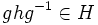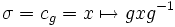# Equivalence of definitions of transitively normal subgroup

This article gives a proof/explanation of the equivalence of multiple definitions for the term transitively normal subgroup
View a complete list of pages giving proofs of equivalence of definitions

## Statement

The following are equivalent for a normal subgroup$K$ of a group$G$:

1. For any normal subgroup$H$ of$K$,$H$ is a normal subgroup of$G$.
2. For any normal automorphism$\sigma$ of$G$, the restriction of$\sigma$ to$K$ is also a normal automorphism of$K$.

## Definitions used

### Normal automorphism

Further information: Normal automorphism (?)

An automorphism$\sigma$ of a group$G$ is termed a normal automorphism if, for every normal subgroup$N$ of$G$,$\sigma$ restricts to an automorphism of$N$.

## Proof

### (1) implies (2)

Given: A group$G$, a subgroup$K$ of$G$ such that any normal subgroup$H$ of$K$ is also a normal subgroup of$G$.$\sigma$ is a normal automorphism of$G$.

To prove:$\sigma$ restricts to an automorphism$\sigma'$ of$K$ that is a normal automorphism of$K$.

Proof:

1.$K$ is normal in$G$: Since$K$ is a normal subgroup of itself, setting$H = K$ in the condition on$K$ yields that$K$ is normal in$G$.
2.$\sigma$ restricts to an automorphism$\sigma'$ of$K$: This follows from the previous step and the definition of normal automorphism.
3. For any normal subgroup$H$ of$K$,$H$ is a normal subgroup of$G$: This is by assumption.
4. For any normal subgroup$H$ of$K$,$\sigma'$ restricts to an automorphism of$H$: By the previous step,$H$ is normal in$G$, so, by assumption,$\sigma$ restricts to an automorphism of$H$. But since$H \le K \le G$ and the restriction of$\sigma$ to$K$ is$\sigma'$, the restriction of$\sigma$ to$H$ equals the restriction of$\sigma'$ to$H$.

From the last step,$\sigma'$ is a normal automorphism of$K$, completing the proof.

### (2) implies (1)

Given: A group$G$, a subgroup$K$ of$G$ such that every normal automorphism of$G$ restricts to a normal automorphism of$K$.$H$ is a normal subgroup of$K$.

To prove:$H$ is a normal subgroup of$G$, i.e., for any$g \in G$ and$h \in H$,$ghg^{-1} \in H$.

Proof: Let$\sigma = c_g = x \mapsto gxg^{-1}$ be conjugation by$g$ (i.e., an inner automorphism).

1.$\sigma = c_g$ is a normal automorphism of$G$: By the definition of normal subgroup,$c_g$ restricts to an automorphism of every normal subgroup of$G$. Thus,$c_g$ is a normal automorphism of$G$.
2. The restriction of$c_g$ to$K$, which we call$\sigma'$, is a normal automorphism of$K$: This follows from the given data for$K$.
3.$\sigma'$ and hence,$\sigma$, restricts to an automorphism of$H$: Since$H$ is a normal subgroup of$K$, and$\sigma'$ is a normal automorphism of$K$,$\sigma'$ restricts to an automorphism of$H$. Hence,$\sigma$ restricts to an automorphism of$H$.
4.$\sigma(h) \in H$, i.e.,$c_g(h) = ghg^{-1} \in H$: This follows immediately from the previous step.